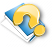## GEOMETRY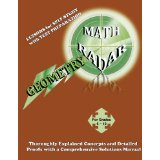Geometry

Geometry covers the following 10 chapters:

• Basic Geometry
• Triangles and Polygons
• Circles
• Geometric Constructions
• Polygonal Regions and their Areas
• Solids and their Volumes, Surface Areas
• Similarity
• The Pythagorean Theorem
• Trigonometric Ratios

## Features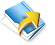Extensive solutions provided

All the mathradar series worksbooks come with exensive solutions manuals, which are either included in the workbook OR sold separately.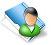Clean and concise description of math concepts

All the MathRadar series workbooks provide a plain and simple explanation of each individual math concept in a way that is easy to understand and follow.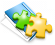Various Examples and solutions for math concepts

Through numerous examples, you will be able to fully understand and develop a sound basis for each math concept, letting you develop confidence in order to assertively approach challenging problems.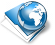Various ways to solve problems

The MathRadar series provides numerous ways to prove each problem, letting you understand the concept more thoroughly and be able to approach difficultly worded problems with strong confidence.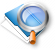Exercises with step-by-step solutions

Each exercise provided in these workbooks has a step-by-step solution and is ranked from easy to hard so you will be able to slowly but steadily work your way through chapters while understanding how to solve and prove.